# Texas Go Math Grade 6 Lesson 15.2 Answer Key Sum of Angle Measures in a Triangle

Refer to our Texas Go Math Grade 6 Answer Key Pdf to score good marks in the exams. Test yourself by practicing the problems from Texas Go Math Grade 6 Lesson 15.2 Answer Key Sum of Angle Measures in a Triangle.

## Texas Go Math Grade 6 Lesson 15.2 Answer Key Sum of Angle Measures in a Triangle

Essential Question
How do you use the sum of angles in a triangle to find an unknown angle measure?

Explore Activity
Exploring Angles in a Triangle
Recall that a triangle is a closed figure with three line segments and three angles. The measures of the angles of a triangle have a special relationship with one another.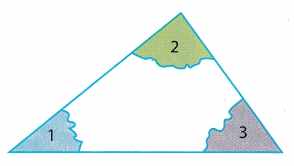A. Use a straightedge to draw a large triangle. Label the angles 1, 2, and 3.

B. Use scissors to cut out the triangle.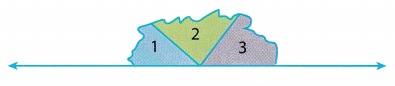C. Tear off the three angles. Arrange them around a point on a line as shown.

D. What is the measure of the straight angle formed by the three angles?

E. What is the sum of the measures of the three angles? Explain.

F. Compare your results with those of your classmates. What guess can you make?

Reflect

Question 1.
Justify Reasoning How can you show that your guess is correct?
When the corner angles of the triangle are placed together as seen on the image, it can form the angle of a straight line Therefore the sum of the inner corner angles in a triangle is equal to 180° which is similar to the angle of a straight angle.
Sum of the inner corner angles is equal to the measurement of the angle in a straight angle.

Find the unknown angle measures.

Question 2.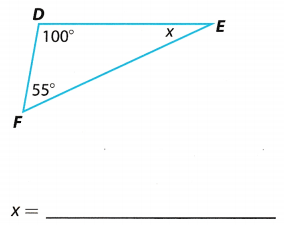Determine the size of the missing angle
55° + 100° + x = 180° substitute for the sum of angle measures in triangle
155° + x = 180° sum of the two angles
155° + x – 155° = 180° – 155° subtract 155° from both sides of the equation
x = 25° measure of the missing angle

The unknown angle measures 25°.

Question 3.Determine the size of the missing angle.
71° + x + 56° = 180° substitute for the sum of angle measures in triangle
127° + x = 180° sum of the two angles
127° + x – 127° = 180° – 127° subtract 127° from both sides of the equation
= 53° measure of the missing angle
The unknown angle is 53°

Example 2.
Find the angle measures in the equilateral triangle.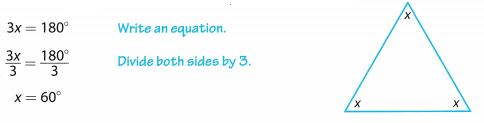Each angle in an equilateral triangle measures 60°.

Reflect

Question 4.
Multiple Representations Write a different equation to find the angle measures in Example 2. Will the answer be the same? Explain.
Different equation for the equilateral triangle.

x + x + x = 180° add all the inner angles in a triangle
3x = 180° sum of all the inner angles
3x = 180°
$$\frac{3 x}{3}$$ = $$\frac{180^{\circ}}{3}$$ divide both sides of the equation by 3
x = 60° value of each angle in an equilateral triangle
The answer is still the same even a different equation was used.

Question 5.
Draw Conclusions Triangle ABC is a right triangle. What conclusions can you draw about the measures of the angles of the triangle?
A right triangle has one angle that measures exactly 90°. If the sum of the angles in a triangle is 180°, then the other two angles must have a total measure of 90°.
The possible measures of the angles can be:
90° + 45° + 45° = 180°
90° + 35° + 55° = 180°
90° + 25° + 65° = 180°
If one angle of a right triangle is a right angle, then the other two angles will, be acute angles.

Write an equation to find the unknown angle measure in each triangle.

Question 6.
The measures of two of the angles are 25° and 65°.
Determine the equation to find the size of the missing angle.
25° + 65° + x = 180°
25° + 65° + x = 180° substitute for the sum of angle measures in triangle
90° + x = 180° sum of the two angles
90° + x – 90° = 180° – 90° subtract 90° from both sides of the equation
x = 90° measure of the missing angle

The equation for the missing angle is 25° + 65° + x = 180° or x = 180° – 90°. The measure of the missing angle is 90°

Question 7.
The measures of two of the angles are 60°.
Determine the equation to find the size of the missing angle.
60° + 60° + x = 180° or x = 180° – 120°
60° + 60° – x = 180° substitute for the sum of angle measures in triangle
120° + x = 180° sum of the two angles
120° + x – 120° = 180° – 120° subtract 120° from both sides of the equation
x = 60° measure of the missing angle

The equation for the missing angle is 60° + 60° + x = 180° or x = 180° – 120°.
The measure of the missing angle is 60°.

Question 8.
The measures of two of the angles are 35°.
Determine the equation to find the size of the missing angle.
35° +x = 180°or x = 180° – 70°
ResuLt
35° + 35° + x = 180° substitute for the sum of angle measures in triangle
70° + x = 180° sum of the two angles
70° + x – 70° = 180° – 70° subtract 70° from both sides of the equation
x = 110° measure of the missing angle
The equation for the missing angle is 35° + 35° + x = 180° or x = 180° – 70°. The measure of the missing angle is 110°.

Question 1.
The sum of the angle measures in a triangle is ______ (Explore Activity)
The sum of the angle measures in a triangle is 180°

When all the inner angles of the triangle are added, the total measurement is 180°

Example 1
Fountain Place, shown to the right, is a 720-foot Dallas skyscraper. Find the measure of the unknown angle in the triangle at the top of the building.
m∠1 + m∠2 + m∠3 = 180° The sum of the angle measures in a triangle is 180°.
65 + 65° + x = 180° Write an equation.
130° + x = 180° Add.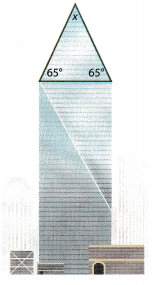x = 50°
The angle at the top of the triangle measures 50°.

Find the unknown angle measure in each triangle. (Examples 1 and 2)

Question 2.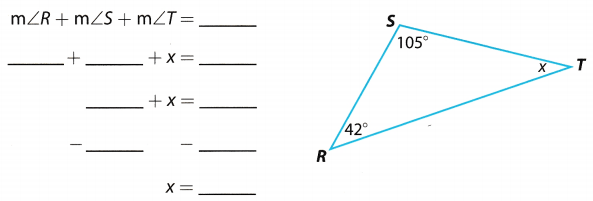Determine the size of the missing angle if the total is 180°.
42° + 105° + x = 180° substitute for the sum of angle measures in triangle
147° + x = 180° sum of the two angles
147° + x – 147° = 180° – 147° subtract 147° from both sides of the equation
x = 33° measure of the missing angle

The missing angle measures 33°.

Question 3.Determine the size of the missing angle.
m∠J + m∠K + m∠L = 180° measures of each angle
x + 96° + 42° = 180° substitute for the sum of angle measures in triangle
x + 138° = 180° sum of the two angles
x + 138° – 138° = 180° – 138° subtract 138° from both sides of the equation
x = 42° measure of the missing angle

The unknown angle measures 42°.

Question 4.Determine the size of the missing angle.

m∠A + m∠B + m∠C = 180° measures of each angle
x + 28° + 90° = 180° substitute for the sum of angle measures in triangle
x + 118° = 180° sum of the two angLes
x + 118° – 118° = 180° – 118° subtract 118° from both sides of the equation
x = 62° measure of the missing angle
The unknown angle measures 62°.

Question 5.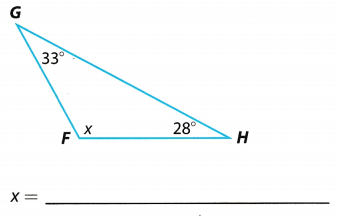Determine the size of the missing angle.
m∠F + m∠G + m∠H = 180° measures of each angle
x + 33° + 28° = 180° substitute for the sum of angle measures in triangle
x + 61° = 180° sum of the two angles
x + 61° – 61° = 180° – 61° subtract 61° from both sides of the equation
x = 119° measure of the missing angle

The unknown angle measures 119°.

Question 6.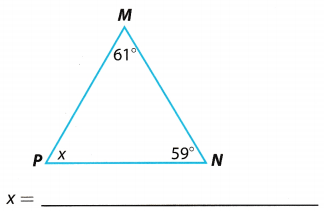Determine the size of the missing angle
m∠M + m∠N + m∠P = 180° measures of each angle
61° + 59° + x = 180° substitute for the sum of angle measures in triangle
120° + x = 180° sum of the two angles
120° + x – 120° = 180° – 120° subtract 120° from both sides of the equation
x = 60° measure of the missing angle

The unknown angle measures 60°.

Question 7.
The measures of two of the angles are 45°. __________
Determine the size of the missing angle.
45° + 45° + x = 180° substitute for the sum of angle measures in triangle
90° + x = 180° sum of the two angles
90° + x – 90° = 180° – 90° subtract 90° from both sides of the equation
x = 90° measure of the missing angle
The unknown angle measures 90°.

Question 8.
The measures of two of the angles are 50° and 30°. ____
Determine the size of the missing angle.
50° + 30° + x = 180° substitute for the sum of angle measures in triangle
80° + x = 180° sum of the two angles
80° + x – 80° = 180° – 80° subtract 80° from both sides of the equation
x = 100° measure of the missing angle

The unknown angle measures 100°.

Essential Question Check-In

Question 9.
Arlen knows the measures of two angles of a triangle. Explain how he can find the measure of the third angle. Why does your method work?
The sum of the angles of a triangle is 180°. If two angles of a triangle are given, he can add the given angles then subtract the sum from 180° to determine the measurement of the third angle.

Add the measurement of the two given angles then subtract from 180° to know the measure of the third angle.

Figure ABCD represents a garden crossed by straight walkway $$\overline{A C} \text {. }$$ Use the figure for 10-15.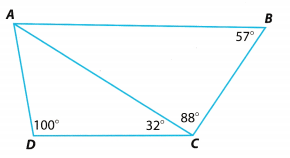Question 10.
Find m∠DAC.
Determine the size of the missing angle.
m∠D + m∠A + m∠C = 180° measures of each angle
100° + x + 32° = 180° substitute for the sum of angle measures in triangle
132° + x = 180° sum of the two angles
132° + x – 132° = 180° – 132° subtract 132° from both sides of the equation
x = 48° measure of the unknown angle
The measure of ∠DAC = 48°

Question 11.
Explain how you found m∠DAC.
The unknown angle is determined by adding the measures of ∠D and ∠C then subtract it from 180°.

Add m∠D and m∠C and subtract the result from 180°.

Question 12.
Find m∠BAC.
Determine the size of the unknown angle.
m∠B + m∠A + m∠C = 180° measures of each angle
57° + x + 88° = 180° substitute for the sum of angle measures in triangle
145° + x = 180° sum of the two angles
145° + x – 145° = 180° – 145° subtract 145° from both sides of the equation
x = 35° measure of the unknown angle

The measure of ∠BAC = 35°.

Question 13.
Explain how you found m∠BAC.
The unknown angle is determined by adding the measures of ∠B and ∠C then subtract it from 180°

Add m∠B and m∠C and subtract the result from 180°.

Question 14.
Find m∠DAB.
Determine the measure of ∠DAB.
m∠DAB = 48° + 35° add the measures of ∠A from ∠DAC and ∠BAC
m∠DAB = 83°
The measure of ∠DAB = 83°.

Question 15.
Explain how you found m∠DAB.
In order to determine the measure of the unknown angle, get the measure of ∠A from ∠DAC and ∠A from ∠BAC. Get the sum of the two angles and that will be the measure of ∠DAB. The indicated angle did not form a triangle therefore, the sum will not be subtracted to 180°.
Add the measures of ∠DAC and ∠BAC.

Question 16.
An observer at point O sees airplane P directly over airport A. The observer measures the angle of the plane at 40.5°.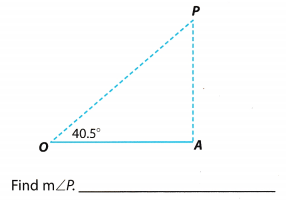Determine the measure of the unknown angle..
m∠O + m∠P + m∠4 = 180° measures of each angle
40.5° + x + 90° = 180° substitute for the sum of angle measures in triangle
130.5° + x = 180° sum of the two angles
130.5° + x – 130.5° = 180° – 130.5° subtract 130.5° from both sides of the equation
x = 49.5° measure of the unknown angle

The measure of ∠P = 49.5°.

The map shows the intersection of three streets in San Antonio’s River Walk district. Use the map for 17-18.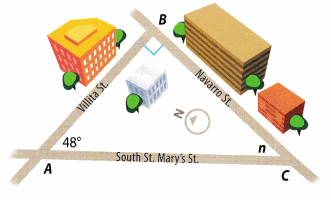Question 17.
Find the measures of the three angles of the triangle.
Determine the measure of the unknown angle.
m∠A + m∠B + m∠C = 180° measures of each angle
48° + 90° + n = 180° substitute for the sum of angle measures in triangle
138° + n = 180° sum of the two angles
138° + n – 138° = 180° – 138° subtract 138° from both sides of the equation
x = 42° measure of the unknown angle

The measures of the three angles are as follows:
m∠A = 48° as indicated on the map
m∠B = 90° because the angle forms a right angle which measures 90°
m∠C = 42° based from the solution

Question 18.
Explain how you found the angle measures.
Measurement of angles are indicated in the figure. However, there are instances when the exact value is not shown but some symbols will describe its measurement. In the given map, the measure of ∠A is already indicated, but the others are not. For ∠B, the small square corner represents a right angle thus the measure is 90°. To get the measure of ∠C, add the measures of the two angles then subtract the sum from 180°

The measure of ∠C can be determined by getting the sum of ∠A and ∠B and subtract the result from 180°.

Texas Go Math Grade 6 Lesson 15.2 H.O.T. Focus On Higher Order Thinking Answer Key

Question 19.
Persevere in Problem Solving Find the measure of ∠ACB. Explain how you found your answer.Determine the inner angle of ∠B from the straight angle which measures 180°.
180° – 148° = 32° measure of the inner angle ∠B
Find the unknown angle.
m∠A + m∠B + m∠C = 180° measures of each angle
83° + 32° + x = 180° substitute for the sum of angle measures in triangle
115° + x = 180° sum of the two angles
115° + x – 115° = 180° – 115° subtract 115° from both sides of the equation
x = 65° measure of the unknown angle

The unknown angle measures 65°.

Question 20.
Communicate Mathematical Ideas Explain how you can use the figure to find the sum of the measures of the angles of quadrilateral ABCD. What is the sum?